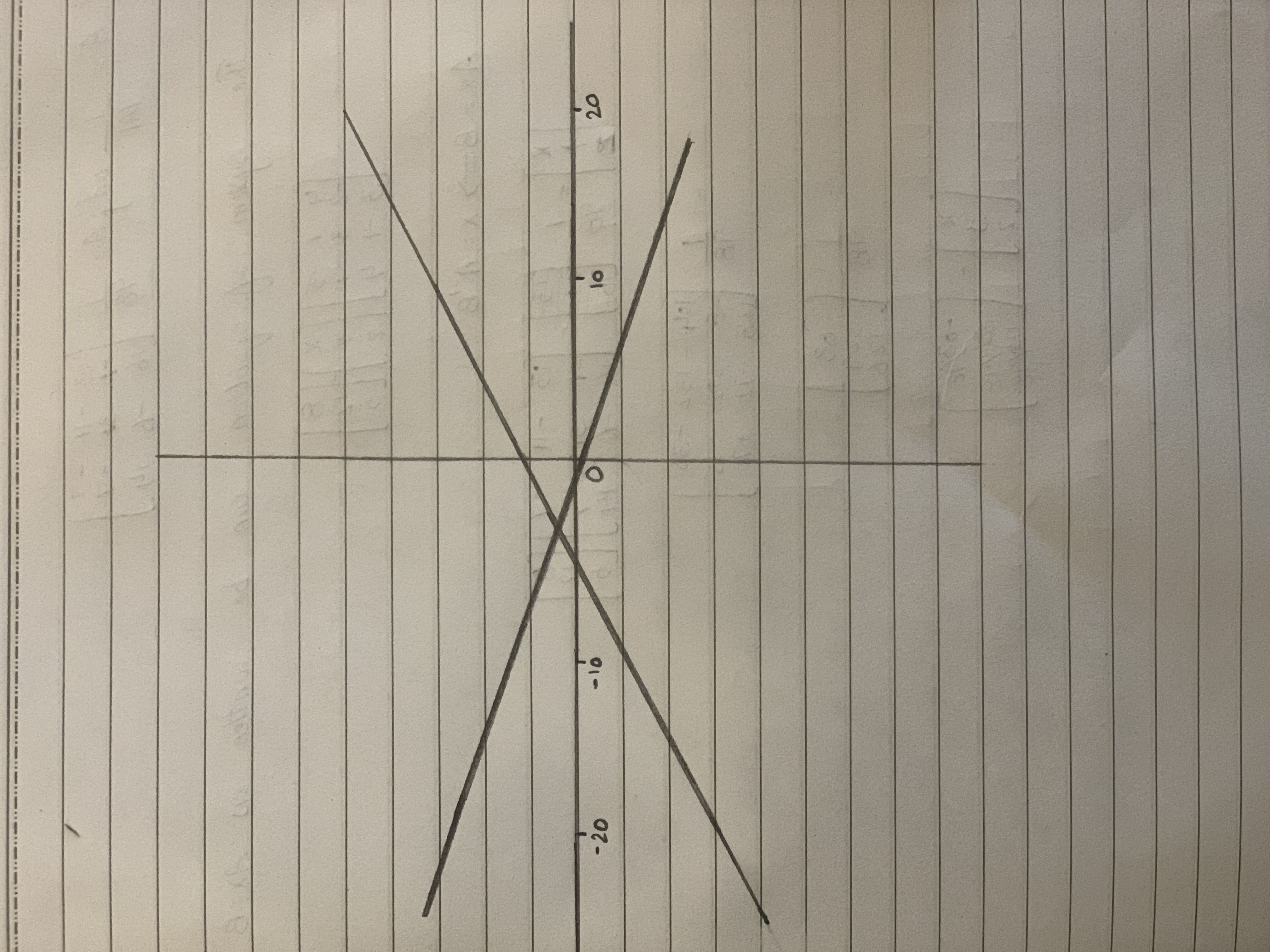# Recognize the equation and important characteristics of the different types of conic sections, illustrate systems of nonlinear equations, determine thopatovaL 2021-01-07 Answered
Recognize the equation and important characteristics of the different types of conic sections, illustrate systems of nonlinear equations, determine the solutions of system of equations (one linear and one second degree) in two variables using substitution, elimination, and graphing (in standard form), solve situational problems involving systems of non-linear equation
Write the following equation in standard form and sketch its
You can still ask an expert for help

• Questions are typically answered in as fast as 30 minutes

Solve your problem for the price of one coffee

• Math expert for every subject
• Pay only if we can solve itpierretteA

Given equation is $9x2+72x-64y2+128y+80=0$.
Given equation can be written as:
$9{x}^{2}+72x-64{y}^{2}+128y+80=0$
$\left(3x{\right)}^{2}+2\ast 3x\ast 12+\left(12{\right)}^{2}-144-\left[64{y}^{2}-128y\right]+80=0$
$\left(3x+12{\right)}^{2}-\left(64{y}^{2}-{128}^{y}+64-64\right)+80-144=0$
$\left(3x+12{\right)}^{2}-\left(8y-8{\right)}^{2}=0$
Given equation is pair of straight lines.